Definitions of Square Dance Calls and Concepts

Index -->  Plus  |  A1  |  A2  |  C1  |  C2  |  C3A  |  C3B  |  C4  |  NOL  |
Definitions (Text Only) -->  Plus  |  A1  |  A2  |  C1  |  C2  |  C3A  |  C3B  |  C4  |  NOL  |
 Find call:

\$BJ?9T\$J(B Line, Column, \$B\$^\$?\$OB>\$NE,@Z\$J(B formation \$B\$+\$i(B.

1. Centers \$B\$O(B Counter Rotate 1/4 \$B\$r(B, Ends \$B\$O(B Concentric 1/2 Zoom \$B\$r\$7\$F(B (\$BIaDL\$N(B) Hinge \$B\$r\$7(B;
2. Centers \$B\$O(B Reverse Flip (Center \$B\$+\$iN%\$l\$k\$h\$&\$K(B Phantom \$B\$H(B Run) \$B\$r(B, Ends \$B\$O(B Phantom Columns Circulate twice \$B\$r\$7\$^\$9(B.

T-Bone \$B\$G\$J\$\$(B 2 x 4 \$B\$+\$i\$OJ?9T\$J(B Line \$B\$G=*\$o\$j\$^\$9(B. Disband \$B\$O(B 2 \$B%Q!<%H\$N%3!<%k\$G\$9(B.

\$BCmDisband \$B\$O(B Detour \$B\$r\$7\$F(B Expand The Column \$B\$HF1\$8\$G\$9(B.

Parallel Waves \$B\$+\$i(B: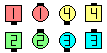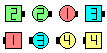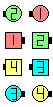Disband\$B\$NA0(B Detour\$B\$N8e(B Expand The Column\$B\$N8e(B (\$B=*\$o\$j(B)
Columns \$B\$+\$i(B: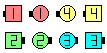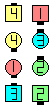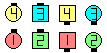Disband\$B\$NA0(B 1/2\$B\$N8e(B Expand The Column\$B\$N8e(B (\$B=*\$o\$j(B)

Detour [C2] (Lee Kopman 1975): Ends \$B\$,(B Tandem \$B\$K\$J\$C\$F\$\$\$kJ?9T\$J(B Line \$B\$+\$i(B, \$B\$^\$?\$O(B Ends \$B\$,(B Tandem \$B\$K\$J\$C\$F\$\$\$kB>\$N(B formation \$B\$+\$i(B. Outsides \$B\$O(B 1/2 Zoom & Hinge \$B\$r\$7(B, Centers \$B\$O(B Counter Rotate 1/4 \$B\$r\$7\$^\$9(B. \$BJ?9T\$J(B Line \$B\$+\$i\$O(B Column \$B\$G=*\$o\$j\$^\$9(B.

Disperse [obsolete] (Lee Kopman 1984): Disband \$B\$HF1\$8\$G\$9\$,(B Centers \$B\$O(B Reverse Flip \$B\$NBe\$o\$j\$K(B Reverse Cut (Trade & Spread) \$B\$r\$7\$^\$9(B.

\$BExpand The Column [C3A].Choreography for DisbandComments? Questions? Suggestions?

https://www.ceder.net/def/disband.php?level=C3B&language=japan
02-October-2022 00:35:55